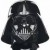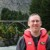# Normal distribution

Six Sigma – iSixSigma Forums Old Forums General Normal distribution

Viewing 12 posts - 1 through 12 (of 12 total)
• Author
Posts
• #45781Hardik
Participant

Hi Team ,
I want to know , why there is so much stress on normality , statement like ” data should be normally distributed ” is there .
By going word ” normality ” following question arises

Why Normality is so much in discussion ,
What is ” Normally distributed data “
If possible sujject me some example to understand this concept
Will appreciate if some one guide me on this thanks Hardik

0
#150370Robert Butler
Participant

You have asked a very broad question and the answer would take far more space and time than a forum of this type would allow.  I’d recommend doing some reading both at the library and on the web.
In an effort to point you in the right direction with respect to your first bullet point I’ve quoted excerpts from pp.72-76 of the section “Adequacy of Normal Distribution as a Physical Model from the book cited below.
“The normal is the most widely used of all distributions. For a long time its importance was exaggerated by the misconception that it was the underlying distribution of nature, and that according to the “Theory of Errors” it was supposed to govern all measurements. With the advent of statistical tests about the year 1900, this assumption was shown not to be universally valid.
Instead, the theoretical justification for the role of the normal distribution is the (or more appropriately “a” since there is more than one) central limit theorem, one of the most important result of mathematical statistics. This theorem states that the distribution of the mean of n independent observations from any distribution, or even from up to n different distributions, with finite mean and variance approaches a normal distribution as the number of observations in the sample becomes large – that is, as n approaches infinity. The result holds, irrespective of the distribution of each of the n elements making up the average.
Although the central limit theorem is concerned with large samples, the sample mean tends to be normally distributed even for relatively small n as long as no single element or small group of elements has a dominating variance and the element distributions do not deviate extremely from a normal distribution.
When a random variable represents the total effect of a large number of independent “small” causes, the central limit theorem thus leads us to expect the distribution of that variable to be normal. Furthermore, empirical evidence has indicated that the normal distribution provides a good representation for many physical variables. The normal distribution has the further advantage for many problems that it is tractable mathematically. Consequently, many of the techniques of statistical inference, such as the method known as the “analysis of variance,” have been derived under the assumption that the data come from a normal distribution.
Because of the prominence, and perhaps the name, of the normal distribution, it is sometimes assumed that a random variable is normally distributed unless proven otherwise. Therefore it should be clearly recognized that many random variables cannot be reasonably regarded as the sum of many small effects, and consequently there is no theoretical reason for expecting a normal distribution. This could be the case when one nonnormal effect is predominant.
The errors of incorrectly assuming normality depend upon the use to which this assumption is put. Many statistical methods derived under this assumption remain valid under moderate deviations and are thus said to be robust. The analysis of variance is an example of a method that is robust under deviations from normality. On the other hand, if the normality assumption were used incorrectly in such problems as determining the proportion of manufactured items above or below some extreme design limit at the tail of the distribution, serious errors might result.”
– From Statistical Models in Engineering – Hahn and Shapiro -1968

0
#150371Ken Feldman
Participant

Robert, you’re still the MAN going into 2007. Look forward to learning a lot from your posts.

0
#150372Chris Seider
Participant

Hardik,
My fellow posters did a nice post but let me answer your first question.  There is so much stressing of making the decision if your data is from a normal distribution or not because the statistical tools vary in their selection depending on the type of distribution.

0
#150373Hardik
Participant

Hi Robert Butler
thanks for gr8 information , do you know any good web url on basic stats .
regards
rahul

0
#150375i am lazy
Participant

do some research and google normal distribution…you’ll be surprised what you come up with.

0
#150377qualityengineer
Participant

Dear all,
Can anbody give some information about where to find about the concept of “distributions”, what it means and from where they have been arisen?

0
#150378Iain Hastings
Participant

It took me longer to write this post than it did to find the link. More distributions than you can shake a stick at.
http://en.wikipedia.org/wiki/Probability_distribution

0
#150381

0
#150396

Excellent.  The only error you make is the transform.  These are not necessary and can make analysis more difficult.  I have a lovely example of a time based histogram for a help desk, bimodal and skewed right, which when tranformed becomes bimodal and skewed left.
You would enjoy reading “Normality and the Process Behaviour Chart” by Wheeler.  A wonderful little book.

0
#150398

The Central Limit Theorem was of interest to Shewhart but he did not use it in formulating control charts.  This has been the source of much misunderstanding, particularly by authors such as Montgomery.
There is no need for the CLT in practical process improvement.

0
#150612Hardik
Participant

Thanks
C Seider , i will keep , u r sujjestion , while doing data analysis .

Rahul

0
Viewing 12 posts - 1 through 12 (of 12 total)

The forum ‘General’ is closed to new topics and replies.